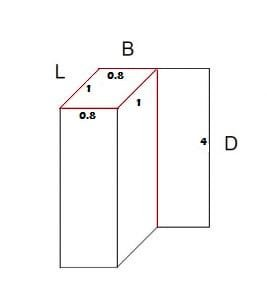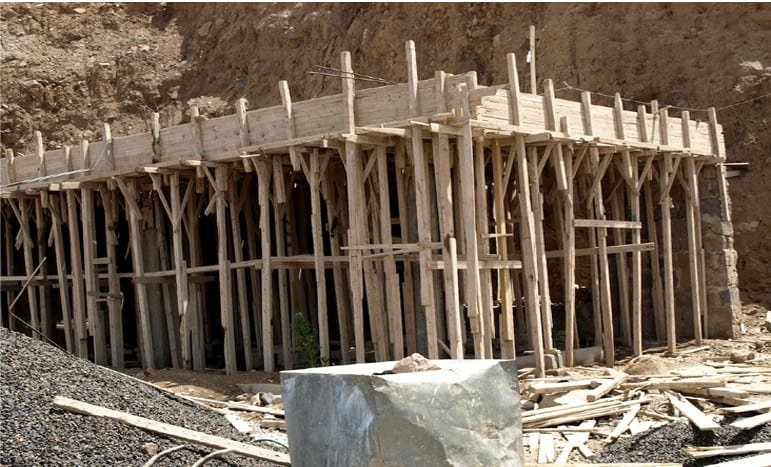## Register Now

Lorem ipsum dolor sit amet, consectetur adipiscing elit.Morbi adipiscing gravdio, sit amet suscipit risus ultrices eu.Fusce viverra neque at purus laoreet consequa.Vivamus vulputate posuere nisl quis consequat.

## How To Calculate Shuttering Area:

Shuttering or formwork is a temporary, rigid structure in which the cast in situ concrete is poured for casting the members to required shape. Different formworks are used for different shape members. In this article, we will discuss how to calculate the shuttering area for construction.

Before calculating the shuttering area first, we need to know how to calculate the peripheral length of any shape.

## Peripheral Length/Perimeter:

We know that perimeter is the distance around a 2D (two dimensional) shape.

For example, square has four sides. Let ‘s’ be the length of one side, then

The peripheral length = s+s+s+s = 4s

Rectangle has four sides also but the length and breadth is different, let one side length is ‘l’ and breadth is ‘b’

Peripheral length = l+b+l+b = 2l+2b

Now coming to the calculation

Shttering area = Peripheral length x Depth

Let me give you an examplePheripheral length = (2 x 1) + (2 x 0.8) = 2 +1.6 =3.6

Total area of shuttering  = 3.6 x 4 = 14.4 sq.m

##Do you have any questions? Let me know in the comments below.